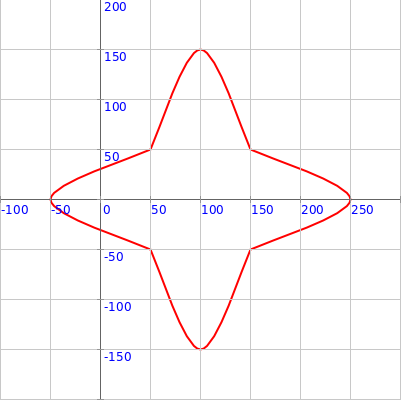## Drawing Vertex based shapes

This activity has the following desired goals:

• Learning to draw shapes using vertices at (x, y) cordinates (A, M).
• Learning to draw curves using vertices at (x, y) cordinates (A, M).
• Using the above ideas to make interesting curved figures (M, T).

### Step 1

Type in the following code and run it:

``````clear()
showAxes()
showGrid()

beginShape()
vertex(50, 50)
vertex(100, 50)
vertex(100, 100)
endShape()
``````

Q1a. Read through the code above and try to understand what it does. What does the above code do? How does it do it?

Q1b. What does the `vertex(x, y)` command do?

### Step 2

Type in the following code and run it:

``````clear()
setSpeed(fast)
showAxes()
showGrid()

beginShape()
curveVertex(50, 50)
curveVertex(50, 50)
curveVertex(150, 150)
curveVertex(250, 50)
curveVertex(250, 50)
endShape()
invisible()
``````

Q2a. Read through the code above and try to understand what it does. What does the above code do? How does it do it?

Q2b. What does the `curveVertex(x, y)` command do?

Q2c. How many `curveVertex(x, y)` calls are required to make a curve?

Now type in the following code and run it:

``````clear()
setSpeed(fast)
showAxes()
showGrid()

beginShape()
curveVertex(0, 200)
curveVertex(50, 50)
curveVertex(150, 150)
curveVertex(250, 50)
curveVertex(300, 50)
endShape()
invisible()
``````

Q2d. Do the first and last `curveVertex(x, y)` calls play a special role in defining the curve to be made?

### Explanation

Command Descriptions:

• `beginShape()` - begins a shape made out of vertices.
• `vertex(x, y)` - adds a vertex to the current shape at the given `(x, y)` location.
• `curveVertex(x, y)` - adds a curve vertex to the current shape at the given `(x, y)` location.
• `endShape()` - finishes the current shape and draws it out.

### Exercise

1 Write a program to make the following figure using one (`beginShape` plus `vertex`es plus `endShape`) shape:2 Write a program to make the following figure using four different (`beginShape` plus `curveVertex`es plus `endShape`) shapes: